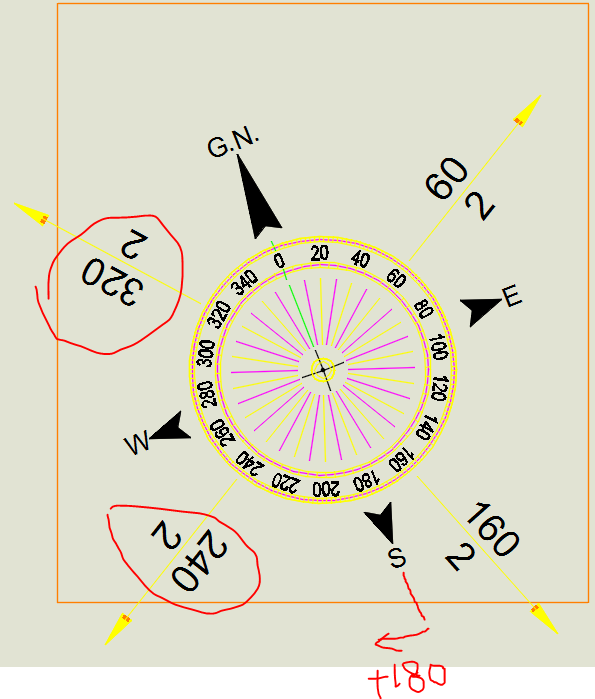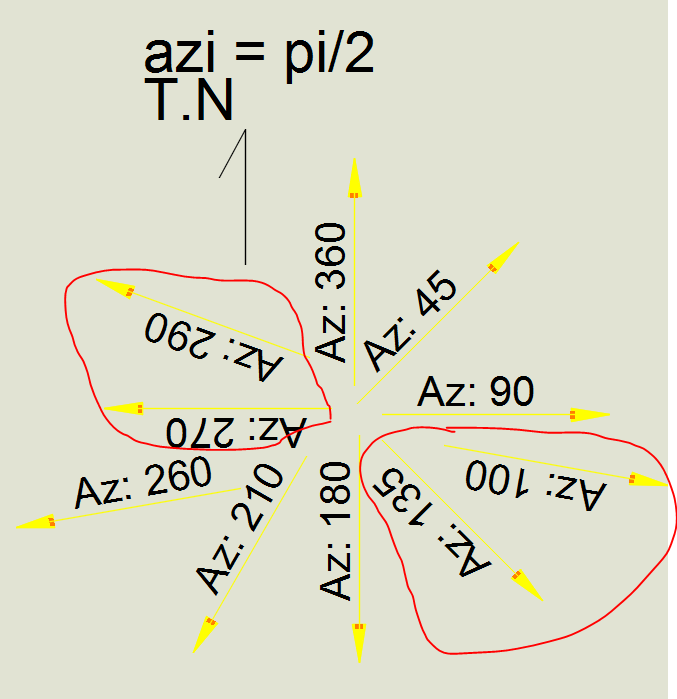# calling a variable from another lisp

## Recommended Postsis there an easy way to call a variable value from another lisp - I googled this and there are varieties of answers that I do not understand.

If it is possible, I would like to call variables azi, eebb and deee from this lisp into other lisps.

When I set my true north to be say: 80 degrees, I would like to take this already set value on this lisp and create another lisp to do (- azi newvaliable) for example.

```(defun c:azi ()
(setq temperr *error*)
(setq *error* trap1)
(if (not azi)(setq azi 0.00))
(setq eebb (getangle (strcat "\nTrue north Direction <" (angtos azi) ">:")))

(if (not eebb)(setq eebb azi)(setq azi eebb))
(setq deee (/ eebb 2))

(princ)
) ;defun```

##### Share on other sitesSince your variables are global (as you have not declared them as local to the function c:azi), they will be defined within the document namespace and will therefore be accessible by any other AutoLISP program whilst the drawing remains open.

Here is a basic example to demonstrate this principle:

```(defun c:test1 ( )
;; Variable 'x' is global
(setq x (getint "\nEnter a number for variable 'x': "))
(princ)
)

(defun c:test2 ( )
(if (null x)
(princ (strcat "\nx * 2 = " (itoa (* x 2))))
)
(princ)
)
```

•1

##### Share on other sitesThank you Lee Mac,

Now I know that is what it means by global vs local: anything declared () would be local to the lisp you execute!

Thank you

##### Share on other sitesCould someone please explain how I should Place the value I get on angD variable to replace the "1" on my leader? if you explain how you obtain the DXF and how you deal with it with some description it would be excellent.

```(defun c:azi (/ osm clay e dee pt2 sel1)

(setq temperr *error*)
(setq *error* trap1)

(if (not azi)(setq azi 0.00))
(setq eebb (getangle (strcat "\nTrue north Direction <" (angtos azi) ">:")))

(if (not eebb)(setq eebb azi)(setq azi eebb))
(setq deee (/ eebb 2))

(princ)
) ;defun

(defun c:IR ( / centro angR Fr obj)

(setq centro (getpoint "\nSelect Block Insertion Point : ")
angD (getreal "\nEnter Rotation Angle for Block in Degrees : ")
angR (* pi (/ angD 180.0))
angF (- azi angR)
);end_setq
(command "_-insert" "r" "_s" "1" centro (angtos angF))
(setq obj (vlax-ename->vla-object (entlast)))
(if (< (/ pi 2) angD (* (/ pi 2) 3))
(foreach prop (vlax-invoke obj 'getdynamicblockproperties)
(if (= (strcase (vla-get-propertyname prop)) "Angle1")
(vla-put-value prop (vlax-make-variant 180.0 (vlax-variant-type (vla-get-value prop))))
);end_if
);end_foreach
);end_if
(princ)
);end_defun
(princ)```

##### Share on other sites4 hours ago, Sambuddy said:

Could someone please explain how I should Place the value I get on angD variable to replace the "1" on my leader? if you explain how you obtain the DXF and how you deal with it with some description it would be excellent.

Consider the following code. I commented out your original code. I am using Visual lisp to insert the block, keep the object in the "blk" variable, then get the array of attributes. Then I use your code block for the dynamic property value. (note: If you want to make it easier to understand - a hint: you can use the "vlax-invoke" code to get a list of attribute objects instead of a safearray - just like you did with the 'GetDynamicBlockProperties method).

```(defun c:azi (/ osm clay e dee pt2 sel1)

(setq temperr *error*)
(setq *error* trap1)

(if (not azi)(setq azi 0.00))
(setq eebb (getangle (strcat "\nTrue north Direction <" (angtos azi) ">:")))

(if (not eebb)(setq eebb azi)(setq azi eebb))
(setq deee (/ eebb 2))

(princ)
) ;defun

(defun c:IR ( / alen atts att blk cnt doc space ts centro angD angR angF obj)

(setq centro (getpoint "\nSelect Block Insertion Point : ")
angD (getreal "\nEnter Rotation Angle for Block in Degrees : ")
angR (* pi (/ angD 180.0))
angF (if azi (- azi angR) angR)
);end_setq
;;   (command "_-insert" "r" "_s" "1" centro (angtos angF))

space (if (> (getvar "CVPORT") 1)(vla-get-modelspace doc)(vla-get-paperspace doc))
blk (vla-insertblock space (vlax-3D-point centro) "r" 1000.0 1000.0 1000.0 angf)
; Note: this method does not account for block units so I had to use 1000 for scale factor to match your drawing.
)

(if blk
(progn
(setq atts (vlax-variant-value (vla-getattributes blk))
alen (vlax-safearray-get-u-bound atts 1)
cnt 0
)
(repeat alen
(setq att (vlax-safearray-get-element atts cnt)
ts  (vla-get-tagstring att)
cnt (1+ cnt)
)
(if (= ts "R")(vla-put-textstring att angD))
)
(if (< (/ pi 2) angD (* (/ pi 2) 3))
(foreach prop (vlax-invoke blk 'getdynamicblockproperties)
(if (= (strcase (vla-get-propertyname prop)) "Angle1")
(vla-put-value prop (vlax-make-variant 180.0 (vlax-variant-type (vla-get-value prop))))
);end_if
);end_foreach
)
)
)
;; 	(setq obj (vlax-ename->vla-object (entlast)))
;; 	  (if (< (/ pi 2) angD (* (/ pi 2) 3))
;;           (foreach prop (vlax-invoke obj 'getdynamicblockproperties)
;;             (if (= (strcase (vla-get-propertyname prop)) "Angle1")
;;               (vla-put-value prop (vlax-make-variant 180.0 (vlax-variant-type (vla-get-value prop))))
;;             );end_if
;;           );end_foreach
;;   );end_if
(princ)
);end_defun
(princ)```

Edited by pkenewell

##### Share on other sitesGood morning Pkenewell,

Thank you very much for your help.

On which line could I add (strcat "Az.: "(angD) "°") for the text would show something like: Az.: 230°? I did try to manipulate a couple of lines but did not succeed.

Also below was my attempt in rotating/ flipping the text so it is always visible but did not succeed. Could you let me know what may be wrong? having in mind that the start degree (0 degree) is from G.N. the reason I use Azi Command (to zero from north) then I simply add angD to it. although I can flip it with controllers on the block, do you think there is a way to rotate them 180 so they are always readable?

Thanks

```(if (< (/ pi 2) angD (* (/ pi 2) 3))
(foreach prop (vlax-invoke blk 'getdynamicblockproperties)
(if (= (strcase (vla-get-propertyname prop)) "Angle1")
(vla-put-value prop (vlax-make-variant 180.0 (vlax-variant-type (vla-get-value prop))))
);end_if
);end_foreach
)
)
)```##### Share on other sitesFor your first part change the following:

`(if (= ts "R")(vla-put-textstring att angD))`

to:

`(if (= ts "R")(vla-put-textstring att (strcat "Az: " (rtos angD))))`

For the second part - the angle needs to be converted to Radians, so use angR instead of angD. Don't have time to test but pretty sure that's it.

`(if (< (/ pi 2) angR (* (/ pi 2) 3)) ; change angD to angR`

Sorry I didn't see that before.

EDIT: Changed (angtos.. to (rtos.. in the first change since "angD" is already in degrees.

Edited by pkenewell

##### Share on other sitesGreat Job as always!

Thank you very much.

Still no luck with the second part though. I used to make it work in the past but it does not see to work now, hah

Thanks pkenewell

##### Share on other sitesOk I have had a chance to test this and the Rotation Parameter is still not changing no matter what I try. Perhaps someone else whom has better knowledge of dynamic blocks can help.

See Solution below in last posted code.

Edited by pkenewell

##### Share on other sitesThanks pkenewell

##### Share on other sitesHa! Figured it out and simple! (2) problems: 1) "Angle1" needed to be in UPPDERCASE, and 2) Parameter angle needed to be in RADIANS. See my updated code:

```(defun c:IR ( / alen atts att blk cnt doc space ts centro angD angR angF)

(setq centro (getpoint "\nSelect Block Insertion Point : ")
angD   (getreal "\nEnter Rotation Angle for Block in Degrees : ")
angR   (* pi (/ angD 180.0))
angF   (if azi (- azi angR) angR)
);end_setq

space (if (> (getvar "CVPORT") 1)(vla-get-modelspace doc)(vla-get-paperspace doc))
blk (vla-insertblock space (vlax-3D-point centro) "r" 1000.0 1000.0 1000.0 angf)
)

(if blk
(progn
(setq atts (vlax-variant-value (vla-getattributes blk))
alen (vlax-safearray-get-u-bound atts 1)
cnt 0
)
(repeat alen
(setq att (vlax-safearray-get-element atts cnt)
ts  (vla-get-tagstring att)
cnt (1+ cnt)
)
(if (= ts "R")(vla-put-textstring att (strcat "Az: " (rtos angD))))
)
(if (< (/ pi 2) (if (< angR 0) (+ angR (* 2 pi)) angR) (* pi 1.5))
(foreach prop (vlax-invoke blk 'getdynamicblockproperties)
(if (= (strcase (vla-get-propertyname prop)) "ANGLE1") ; Figured it out! Param name needs to be in UPPERCASE
(vla-put-value prop (vlax-make-variant pi (vlax-variant-type (vla-get-value prop)))); Value for 180 in Radians
);end_if
);end_foreach
)
)
)
(princ)
);end_defun```

##### Share on other sitesWell done! have a bit of a problem with 2nd quarter and 4th quarter though.

but VERY NICE touch pkenewell##### Share on other sitesAnother Update: I think the problem lies in that the angle to test should be the "angF" variable. Also need to keep the angle positive (if neg - add 2pi).

FYI: I updated your AZI command too to be more efficient and use a better global variable

```(defun c:AZI ()
(if (not IR:AZI)(setq IR:AZI 0.0))
(setq IR:AZI
(cond
((getangle (strcat "\nTrue north Direction <" (angtos IR:AZI) ">:")))
(IR:AZI)
)
)
(princ)
)

(defun c:IR ( / alen atts att blk cnt doc space ts centro angD angR angF)

(setq centro (getpoint "\nSelect Block Insertion Point : ")
angD   (getreal "\nEnter Rotation Angle for Block in Degrees : ")
angR   (* pi (/ angD 180.0))
angF   (if IR:AZI (- IR:AZI angR) angR)
);end_setq

space (if (> (getvar "CVPORT") 1)(vla-get-modelspace doc)(vla-get-paperspace doc))
blk (vla-insertblock space (vlax-3D-point centro) "r" 1000.0 1000.0 1000.0 angf)
)

(if blk
(progn
(setq atts (vlax-variant-value (vla-getattributes blk))
alen (vlax-safearray-get-u-bound atts 1)
cnt 0
)
(repeat alen
(setq att (vlax-safearray-get-element atts cnt)
ts  (vla-get-tagstring att)
cnt (1+ cnt)
)
(if (= ts "R")(vla-put-textstring att (strcat "Az: " (rtos angD))))
)
(if (< (/ pi 2) (if (< angF 0) (+ angF (* 2 pi)) angF) (* pi 1.5)); Check positive angF value
(foreach prop (vlax-invoke blk 'getdynamicblockproperties)
(if (= (strcase (vla-get-propertyname prop)) "ANGLE1") ; Figured it out! Param name needs to be in UPPERCASE
(vla-put-value prop (vlax-make-variant pi (vlax-variant-type (vla-get-value prop)))); 180 in Radians
);end_if
);end_foreach
)
)
)
(princ)
);end_defun
(princ)```

I tested this and it works for all angles.

Edited by pkenewell

##### Share on other sitesEdited by BIGAL

##### Share on other sitesI managed to rotate them using the lisp below because I quit on my initial thoughts but pkenewell proved he is another creature. I have been scratching my head for a while on this rotating depending on the difference between two angles for a while and he solved it so casually  - Both pkenewell and BIGAL must be alians (super advanced) (in a good sense hah)!

Thank you again pkenewell

```(DEFUN C:IT (/ BS BSL CT NOFUV NOFNU LP NE NEL NEAET NEA NEAS OFNU NNEL)
(PROMPT "\n*SET ATTRIBUTE ROTATION* ")
(PROMPT "\nSelect block with attributes to rotate: ")
(SETQ BS (SSGET '((-4 . "<AND") (0 . "INSERT") (66 . 1) (-4 . "AND>")) ))
(SETQ BSL (SSLENGTH BS))
(SETQ CT (- BSL 1))
(SETQ NOFUV (GETANGLE "\nNew attribute rotation angle: "))
(SETQ LP 1)
(WHILE LP
(SETQ NE (SSNAME BS CT))
(SETQ CT2 0)
(SETQ LP2 1)
(WHILE LP2
(SETQ NE (ENTNEXT NE))
(SETQ NEL (ENTGET NE))
(SETQ NEAET (CDR (ASSOC 0 NEL)))
(SETQ NEA (ASSOC 50 NEL))
(SETQ NEAS (CDR NEA))
(IF (= NEAET "ATTRIB")
(PROGN
(IF (/= NEA NIL)
(PROGN
(SETQ NOFNU (CONS 50 NOFUV))
(SETQ OFNU NEA)
(SETQ NNEL (SUBST NOFNU OFNU NEL))
(ENTMOD NNEL)
(ENTUPD NE)
));END PROGN/IF NEA
));END PROGN/IF NEAET
(IF (= NEAET "SEQEND") (SETQ LP2 NIL))
);END WHILE LP2
(SETQ CT (- CT 1))
(IF (< CT 0) (SETQ LP NIL))
);END WHILE LP
(PRINC)
);END DEFUN```

I am sure even this lisp has a lot of holes in it!

Edited by Sambuddy
•1

## Join the conversation

You can post now and register later. If you have an account, sign in now to post with your account.
Note: Your post will require moderator approval before it will be visible.×   Pasted as rich text.   Paste as plain text instead

Only 75 emoji are allowed.